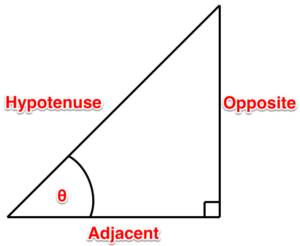# 1.4: Naming Right Triangle Sides

$$\newcommand{\vecs}{\overset { \rightharpoonup} {\mathbf{#1}} }$$ $$\newcommand{\vecd}{\overset{-\!-\!\rightharpoonup}{\vphantom{a}\smash {#1}}}$$$$\newcommand{\id}{\mathrm{id}}$$ $$\newcommand{\Span}{\mathrm{span}}$$ $$\newcommand{\kernel}{\mathrm{null}\,}$$ $$\newcommand{\range}{\mathrm{range}\,}$$ $$\newcommand{\RealPart}{\mathrm{Re}}$$ $$\newcommand{\ImaginaryPart}{\mathrm{Im}}$$ $$\newcommand{\Argument}{\mathrm{Arg}}$$ $$\newcommand{\norm}{\| #1 \|}$$ $$\newcommand{\inner}{\langle #1, #2 \rangle}$$ $$\newcommand{\Span}{\mathrm{span}}$$ $$\newcommand{\id}{\mathrm{id}}$$ $$\newcommand{\Span}{\mathrm{span}}$$ $$\newcommand{\kernel}{\mathrm{null}\,}$$ $$\newcommand{\range}{\mathrm{range}\,}$$ $$\newcommand{\RealPart}{\mathrm{Re}}$$ $$\newcommand{\ImaginaryPart}{\mathrm{Im}}$$ $$\newcommand{\Argument}{\mathrm{Arg}}$$ $$\newcommand{\norm}{\| #1 \|}$$ $$\newcommand{\inner}{\langle #1, #2 \rangle}$$ $$\newcommand{\Span}{\mathrm{span}}$$$$\newcommand{\AA}{\unicode[.8,0]{x212B}}$$

## What is this big fancy word, trigonometry?

Trigonometry is the study of the relationship that exists between the sides and the angles of a triangle.

That sounds complicated and scary.

It can be, but lucky for us we are only dealing with right triangles. This makes it very simple and almost fun. (Nerd alert!)

## First steps

We have already learned how to determine the sides of a triangle using the Pythagoras’ theorem. Next up is using those sides to determine the angles. Lucky for us we know that in a right triangle we already have one 90-degree angle. We also know that if we can solve any of the other two angles, the third one is easy. (All triangles have 180 degrees.) Our next step is to name the sides of the triangle. The names of these sides are dependent on something called the designate angle or theta. Theta is an angle that you determine or is determined for you.Figure 16. Theta 1Figure 17. Theta 2

Now once you have figured out which angle is your theta, we can get to business naming the sides.

Adjacent. This is the side that sits adjacent to the designate angle.

Opposite. This is the side that sits opposite to the designate angle.

Hypotenuse. This is the side that sits opposite the 90-degree angle.

The hypotenuse is always the longest side of the triangle and doesn’t care where the designate angle is. It only cares that it is opposite the 90-degree angle.Figure 18. Hypotenuse

And if we switch the designate angle, the names of the sides change as in Figure 19.Figure 19. Adjacent and Opposite sides switch places

The next chapter tells us what to do with these names.

This page titled 1.4: Naming Right Triangle Sides is shared under a CC BY 4.0 license and was authored, remixed, and/or curated by Chad Flinn (BC Campus) via source content that was edited to the style and standards of the LibreTexts platform; a detailed edit history is available upon request.